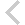FICO Xpress Optimization Examples Repository
 FICO Optimization Community FICO Xpress Optimization HomeUsing NumPy arrays to create variables

Description
Use NumPy arrays for creating a 3-dimensional array of variables, then use it to create a model.

Further explanation of this example: 'Xpress Python Reference Manual'

Source Files
By clicking on a file name, a preview is opened at the bottom of this page.

example_array.py

# Use NumPy arrays for creating a 3-dimensional array of variables,
# then use it to create a mode.
#
# (C) Fair Isaac Corp., 1983-2021

from __future__ import print_function

import numpy as np
import xpress as xp

S1 = range(2)
S2 = range(3)
S3 = range(4)

m = xp.problem()

# Create a NumPy array of variables using the xp.npvar keyword. This
# is to ensure NumPy handles Xpress variable objects.
h = np.array([[[xp.var(vartype=xp.binary) for i in S1]
for j in S2] for k in S3], dtype=xp.npvar)

m.setObjective(h * h +
h * h +
h * h +
xp.Sum(h[i][j][k]
for i in S3 for j in S2 for k in S1))

cons00 = - h * h + \
xp.Sum(i * j * k * h[i][j][k]
for i in S3 for j in S2 for k in S1) >= 11

m.solve()

# Get the matrix representation of the quadratic part of the single
# constraint

mstart1 = []
mclind1 = []
dqe1 = []
m.getqrowqmatrix(cons00, mstart1, mclind1, dqe1, 29,
h, h)
print("row 0:", mstart1, mclind1, dqe1)